# The sum 4

The sum of Robin's age is 45. Seven years ago, Robin was 16 years more than one half as old as Bruno then. How old is Bruno?

B =  17

### Step-by-step explanation:

B+R = 45
(R-7) = 16+(B-7)/2

B+R = 45
B-2R = -39

B = 17
R = 28

Our linear equations calculator calculates it.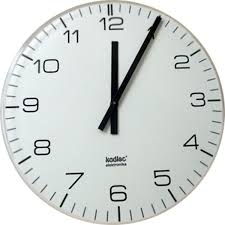Did you find an error or inaccuracy? Feel free to write us. Thank you!Tips to related online calculators
Need help to calculate sum, simplify or multiply fractions? Try our fraction calculator.
Do you have a system of equations and looking for calculator system of linear equations?
Do you want to convert time units like minutes to seconds?

## Related math problems and questions:

• Age problemsA) Alex is 3 times as old as he was 2 years ago. How old is he now? b) Casey was twice as old as his sister 3 years ago. Now he is 5 years older than his sister. How old is Casey? c) Jessica is 4 years younger than Jennifer now. In 10 years, Jessica will
• CarlaCarla is 5 years old, and Jim is 13 years younger than Peter. One year ago, Peter's age was twice the sum of Carla's and Jim's age. Find the present age of each one of them.
• After 16 years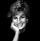After 16 years will Dana be five times older as she was four years ago. After how many years will Dana celebrate her 16th birthday?
• Maria 2Maria is 7 years older than her brother Robert and in 2 years her age will be four times what Robert’s age was three years ago. Find their present ages.
• Lee isLee is 8 years more than twice Park's age, 4 years ago, Lee was three times as old. How old was Lee 4 years ago?
• Speed of Slovakian trainsRudolf decided to take the train from the station 'Ostratice' to 'Horné Ozorovce'. In the train timetables found train Os 5409 : km 0 Chynorany 15:17 5 Ostratice 15:23 15:23 8 Rybany 15:27 15:27 10 Dolné Naštice 15:31 15:31 14 Bánovce nad Bebravou 15:35 1
• Three men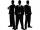Alex is half younger than Jan, which is one-third younger than George. The sum of their ages is 48. How are these three men old?
• Average ageThe company of five people has an average age of 46 years. The average age of the first four is 43 years. How many years has the fifth member of this company?
• Mother and daughter 2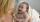The mother is 40 years older than her daughter. How old is the mother if her age is eight thirds age of daughter?
• Two numbersWe have two numbers. Their sum is 140. One-fifth of the first number is equal to half the second number. Determine those unknown numbers.
• The sum 16The sum of A and B is 36. The difference of A & B is 8. find the larger number of A.
• 14 yearsKája is 14 years old. Mom 44. How many years will mom be 4 times older?
• Father and son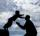Father is 44 years old, his son 16 years. Determine how many years ago was the father five times older than the son.
• Age ratioJanko is 14 years old. The age ratio of Janka and Zuzka is 2: 3. What was the ratio seven years ago?
• Ages 2A man's age is 4 times his son's age. After 5 years he will be just twice his son's age, find their ages.
• AgeIn 1960 my age was equal to the digits sum of the year of my birth. What is my age now?
• Father and sonsAfter15 years will father many years as his two sons together now. There is a six-year difference between the brothers, and the older one celebrated fifty years three years ago. How old is their father now?